## Posts Tagged ‘probability’

### I Need A Speech Bubble To Appear Over My Head When I Talk So I Can Diagram the Bayesian Uncertainty In My Statements

May 2, 2012
April 26, 2012

My formula for success is rise early, work late, and strike oil.

J P Getty, How to Be Rich

April 20, 2012

Mostly in finance we assume that we have the equivalent of a standard dice. That is, while we assume we don’t know what number will come up next, we think that we know the distribution of numbers perfectly.

In fact the real situation is much more akin to throwing a dice where we have imperfect knowledge of what numbers are on the faces. They might be 1 to 6; but they also might be 1 to 5 with the 1 repeated; or 2 to 7; or something else entirely.

Worse, the numbers are changed by the malevolent hand of chance on a regular basis.

Not so often that we know nothing about the distribution, but often enough that we cannot be sure that the current market will be like the past.

David Murphy

(And I would add: sometimes the numbers on the die are being changed not by the malevolent hand of chance, but by the malevolent hand of a market participant who is smarter than you and can siphon your profits into their bank account.)

March 24, 2012

If you put your hand up in the night, away from L.A., and look at a dark spot of the sky the size of a dime—with a large enough telescope, you could see 100,000 galaxies there.

Stars explode once every 100 years per galaxy. So in that little region with 100,000 galaxies, on a given night you’ll see ten stars explode.

The universe is huge, and old, and rare things happen all the time.

Lawrence Krauss

minute 17:30 of this lecture

### How Not To Draw a Probability Distribution

March 7, 2012

If I google for “probability distribution” I find the following extremely bad picture:It’s bad because it conflates ideas and oversimplifies how variable probability distributions can generally be.

• Most distributions are not unimodal.
• Most distributions are not symmetric.
• Most distributions do not have `mean` = `median` = `mode`.
• Most distributions are not Gaussian, Poisson, binomial, or anything famous at all.• If this is the example you give to your students of “a distribution”, why in the world would they be surprised at the Central Limit Theorem? The reason it’s interesting is that things that don’t look like the above, sum to look like the above.
• People already mistakenly assume that everything is bell curved. Don’t reinforce the notion!
` `

Here is a better picture to use in exposition. In `R` I defined

`bimodal <- function(x) { 3 * dnorm(x, mean=0, sd=1)   +   dnorm(x, mean=3, sd=.3) / 4                         }`.

That’s what you see here, plotted with `plot( bimodal, -3, 5, lwd=3, col="#333333", yaxt="n" )`.Here’s how I calculated the mean, median, and mode:

• mean is the most familiar  . To calculate this in `R` I defined `bimodal.x <- function(x) { x * 3 * dnorm(x, mean=0, sd=1)   +   x * dnorm(x, mean=3, sd=.3) / 4  }` and did `integrate(bimodal.x, lower=-Inf, upper=Inf)`.

The output is `.75`, that’s the mean.

• mode is the x where the highest point is. That’s obviously zero. In fancy scary notation one writes “the argument of the highest probability”
• median is the most useful but also the hardest one to write the formulaic definition. Median has 50% of the probability mass to the left and 50% of the probability mass to the right. So In `R` I had to plug in lots of values to `integrate( bimodal, lower = -Inf, upper = ... )` and `integrate( bimodal, upper = Inf, lower = ...)` until I got them to be equal. I could have been a little smarter and tried to make the difference equal zero but the way I did it made sense and was quick enough.

The answer is roughly `.12`.

```> integrate( bimodal, lower = -Inf, upper = .12 )
1.643275 with absolute error < 1.8e-08
> integrate( bimodal, upper = Inf, lower = .12 )
1.606725 with absolute error < 0.0000027```

(I could have even found the exact value in Excel using the solver. But I felt lazy, please excuse me.)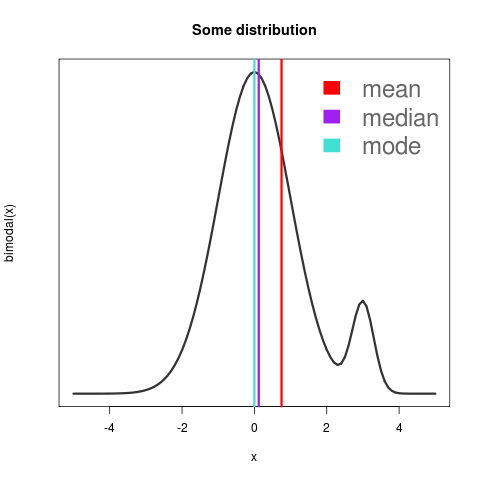Notice that I drew the numbers as vertical lines rather than points on the curve. And I eliminated the vertical axis labels. That’s because the mean, median, and mode are all x values and have nothing whatever to do with the vertical value. If I could have figured out how to draw a coloured dot at the bottom, I would have. You could also argue that I should have shown more humps or made the mean and median diverge even more.

Here’s how I drew the above:

```png("some bimodal dist.png")
leg.text <- c("mean", "median", "mode")
leg.col <- c("red", "purple", "turquoise")
par(lwd=3, col="#333333")
plot( bimodal, -5, 5, main = "Some distribution", yaxt="n" )
abline(v = 0, col = "turquoise")
abline(v = .12, col = "purple")
abline(v = .75, col = "red")
legend(x = "topright", legend = leg.text, fill = leg.col, border="white", bty="n", cex = 2, text.col = "#666666")
dev.off() ```

Lastly, it’s not that hard in the computer era to get an actual distribution drawn from facts. The `nlme` package has actually recorded heights of boys from Oxford:

```require(nlme); data(Oxboys); plot( density( Oxboys\$height), main = "height of boys from Oxford", yaxt="n", lwd=3, col="#333333")
```

and boom:or in histogram form with `ggplot`, run `require(ggplot2); qplot( data = Oxboys, x = height )` and get:the heights look Gaussian-ish, without mistakenly giving students the impression that real-world data follows perfect bell-shaped patterns.

### Partition Function

February 28, 2012

5 = 5
5 = 4 + 1
5 = 3 + 2
5 = 3 + 1 + 1
5 = 2 + 2 + 1
5 = 2 + 1 + 1 + 1
5 = 1 + 1 + 1 + 1 + 1

There are 7 ways to split up five things. Seven different ways you could divide up 5 balls, 5 dolls, 5 wrapped-up candies; seven microstate subsets of a 5-molecule gas.How many ways are there to divide up 4 things? 8 things? 20,000 things? 198^198 things? Even if you had a few days to write a computer program that would brute-force count these things, how would you do it?

I would try to come up with a way to “break one off” from the largest number and count the “leaves” off each branch, somehow trying to remember to go back and “break off two” — and make sure I haven’t forgotten any other contingencies. It wouldn’t be pretty (although perhaps it would be prettier in a functional language).

Here are the first few answers. I don’t see an obvious pattern

• 1, 1, 2, 3, 5, 7, 11, 15, 22, 30, 42, 56, 77, 101, 135, 176, 231, 297, 385, 490, 627, 792, 1002, 1255, 1575, 1958, 2436, 3010, 3718, 4565, 5604, 6842, 8349, 10143, 12310, 14883, 17977, 21637, 26015, 31185, 37338, 44583, 53174, 63261, 75175, 89134` `

The answers to this line of questions—which answers are given by the partition function — come up in weird places en route to the answer to some other question. For example, the partition function comes up in thermodynamics. WTF? Don’t ask me, I didn’t make up the universe, or logic. The partition function also shows its face when you try to reason out measures of statistical validity. That at least makes sense because these partitions are combinatoric in character.

But back to the question—how would you figure out `NumPartitions(1)`, `NumPartitions(2)`, `NumPartitions(3)`, … and so on? Is there a formula for it? Or do you just have to find 20,000 stones and start breaking them up into groups (or simulate such on the computer) to find out `NumPartitions(20,000)`?

Herbert Wilf explains here

that there is a better way to express the outcomes of the Partition Function. (Much better than my program idea.) First you have to know that you can encode sequences as polynomials. Second, recognise that the sequence / polynomial coefficients represent a function from ℕ→ℕ (How many ways to divide up 1? `p(1)` How many ways to divide up 8? `p(8)`. Etc.). It’s called Euler’s generating function. Using the sequence polynomial trick, you can say “The entire sequence of answers to `p(n)` for `n=1` thru `∞` can be written `∑p(n •x^n`.” (Because the polynomial encodes the sequence.)

Third, here is the answer: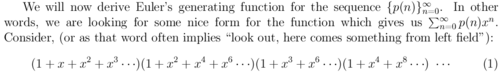Essentially, if you “multiply” together the sequences ` [0,1,2,3,4,5,...] `, `[0,2,4,6,8,...]`, `[0,4,8,12,16,...] `, and so on, you get the appropriate sequence of outputs that answers the partition question.

Wow. First of all, whoever figured this out should be crowned king of innovation for 100 years. Second of all, why is Nature so weird? I mean, this result seems way too simple to be true. Third of all, given what I said about polynomials as sequences, now we’ve established a way to factor a certain sequence (the partition sequence) into products of sequences. I wonder where else you could go with that—either analogies, or tweaking-the-pattern a little bit, or applications of this exact idea to other fields where you wouldn’t normally think to yourself “Here I have a polynomial.”

So. From candies to statistics to number theory to thermodynamics to algebraic rings to who knows how to describe what we have seen here. Such a simple question to ask, with a complicated answer, but somebody has figured out how to make it easy after all. All I can say is, I’m not making this stuff up.

February 11, 2012Leonardo da Vinci’s ability to embrace uncertainty, ambiguity, and paradox was a critical characteristic of his genius. —J Michael Gelb

Say you want to use a mathematical metaphor, but you don’t want to be really precise. Here are some ways to do that:

• Tack a onto the end of an equation.
• Use bounds (“I expect to make less than a trillion dollars over my lifetime and more than \$0.”)
• Speak about a general class without specifying which member of the class you’re talking about. (The members all share some property like, being feminists, without necessarily having other properties like, being women or being angry.)
• Use fuzzy logic (the  membership relation gets a percent attached to it: “I 30%-belong-to the class of feminists | vegetarians | successful people.”).
• Use a specific probability distribution like Gaussian, Cauchy, Weibull.
• Use a tempered distribution a.k.a. a Schwartz function.

Tempered distributions are my favourite way of thinking mathematically imprecisely.

Tempered distributions have exact upper and lower bounds but an inexact mean and variance. T.D.’s also shoot down very fast (like `exp{−x²}` the gaussian) which makes them tractable.

For example I can talk about the temperature in the room (there is not just one temperature since there are several moles of air molecules in the room), the position of a quantum particle, my fuzzy inclusion in the set of vegetarians, my confidence level in a business forecast, ….. with a definite, imprecise meaning.

Classroom mathematics usually involves precise formulas but the level of generality achieved by 20th century mathematicians allows us to talk about a cobordism between two things without knowing everything precisely about them.

It’s funny; the more advanced and general the mathematics, the more casual it can become. Like stingy stickler things that build up to a chummy, whatever-it’s-all-good.

` `

Our knowledge of the world is not only piecemeal, but also vague and imprecise. To link mathematics to our conceptions of the real world, therefore, requires imprecision.

I want the option of thinking about my life, commerce, the natural world, art, and ideas using manifolds, metrics, functors, topological connections, lattices, orthogonality, linear spans, categories, geometry, and any other metaphor, if I wish.

December 4, 2011

The actual science of logic is conversant at present only with things either certain, impossible, or entirely doubtful, none of which (fortunately) we have to reason on.

Therefore the true logic for this world is the calculus of Probabilities, which takes account of the magnitude of the probability which is, or ought to be, in a reasonable man’s mind.

James Clerk Maxwell (1850), quoted in Plausible Reasoning (1994)

October 23, 2011

While the black and white populations of the United States have long differed in various social and economic variables — in income, years of schooling, life expectancy, unemployment rates, crime rates, and scores on a variety of tests — so have other groups differed widely from one another and from the national average in countries and around the world.

It has often been common to compare a given group, such as blacks in the United States, with the national average and regard the differences as showing a special peculiarity of the group being compared, or a special peculiarity of policies or attitudes toward that group. But either conclusion can be misleading when the national average itself is just an amalgamation of wide variations among ethnic, regional, and other groups.

One of the most overlooked, but important, differences among groups are their ages. The median age of black Americans is five years younger than the median age (35) of the American population as a whole, but blacks are by no means unique in having a median age different from the national average or from the ages of other groups.

Among Asian Americans, the median age ranges from 43 for Japanese Americans to 24 for Americans of Cambodian ancestry to 16 for those of Hmong ancestry.

Incomes are highly correlated with age, with young people usually … earning much less than older and more experienced workers.

Thomas Sowell, in Economic Facts and Fallacies### Hey! I made you some Wiener processes!

September 7, 2011

Check them out.

Here are thirty homoskedastic ones:

`> homo.wiener <- array(0, c(100, 30))> for (j in 1:30) {  for (i in 2:length(homo.wiener)) {          homo.wiener[i,j] <-  homo.wiener[ i - 1, j] + rnorm(1)                     }}`

`> for (j in 1:30) {`

`       plot( homo.wiener[,j],           type = "l", col = rgb(.1,.1,.1,.6),          ylab="", xlab="", ylim=c(-25,25)            );             par(new=TRUE) } `` `

Here’s just the meat of that wiener, in case the `for` loops or window dressing were confusing.

`homo.wiener[i] <-  homo.wiener[ i - 1] + rnorm(1)`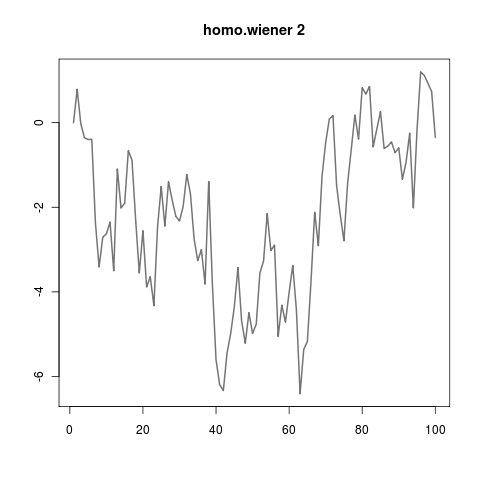` `

I also made you some heteroskedastic wieners.

`> same for-loop encasing. ∀ j make wieners; ∀j plot wieners> hetero.wiener[i] <- hetero.wiener[ i-1 ] + rnorm(1, sd=rpois(1,1) )`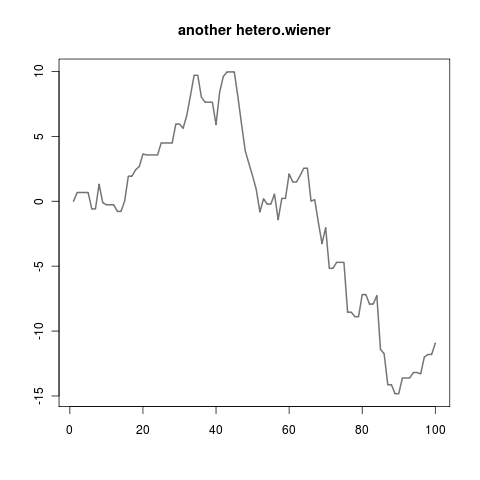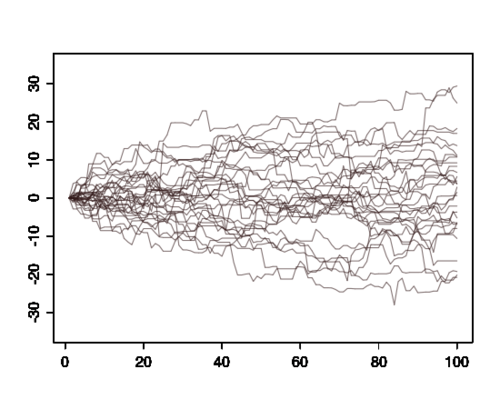` `

It wasn’t even that hard — here are some autoregressive(1) wieners as well.

`> same for-loop encasing. ∀j make wieners; ∀j plot wieners> ar.wiener[i] <- ar.wiener[i-1]*.9 + rnorm(1)`` `

Other types of wieners:

• `a.wiener[i-1] + rnorm(1) * a.wiener[i-1] + rnorm(1)`
• `central.limit.wiener[i-1] + sum( runif(17, min=-1) )`
• `cauchy.wiener[i-1] + rcauchy(1)      #leaping lizards!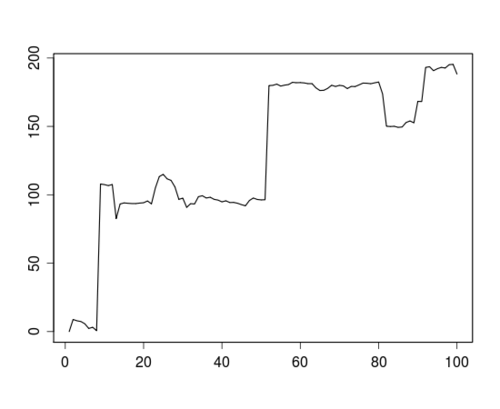`
• `random.eruption.wiener[i-1] + rnorm(1) * random.eruption.wiener[i-1] + rnorm(1)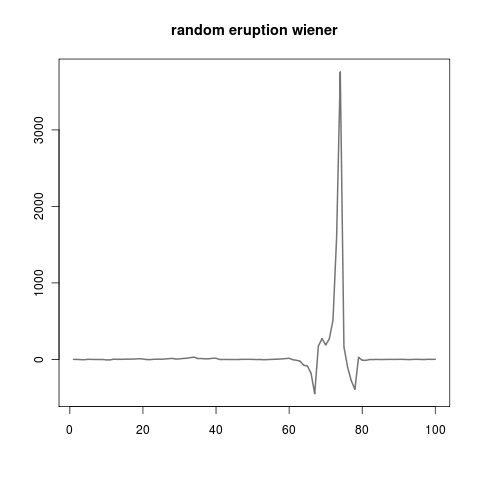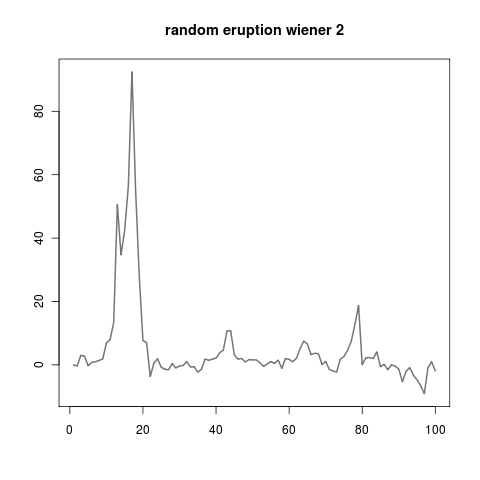`
• `non.markov.wiener[i-1] + non.markov.wiener[i-2] + rnorm(1)`
• `the.wiener.that.never.forgets[i] <- cumsum( the.wiener.that.never.forgets) + rnorm(1)`
• `non.wiener[i] <- rnorm(1)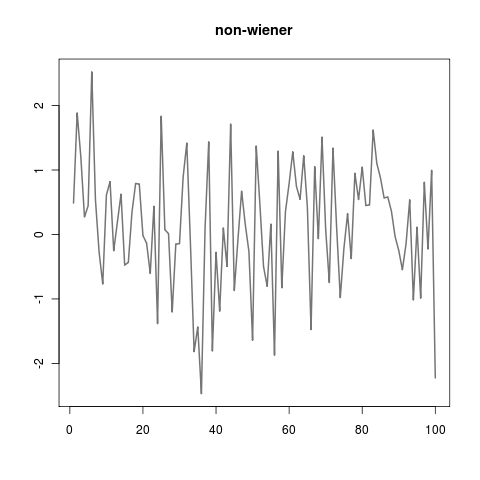`
• `moving.average.3.wiener[i] <- .6 * rnorm(n=1,sd=1) + .1 * rnorm(n=1,sd=50) + .3 * rnorm(n=1, mean=-3,sd=17)`
• `2d.wiener <- array(0, c(2, 100));ifelse( runif(1) > .5,     2d.wiener[1,i] <- 2d.wiener[1,i-1] + rnorm(1)             && 2d.wiener[2,i] <- 2d.wiener[2,i-1],     2d.wiener[2,i] <- 2d.wiener[2,i-1] + rnorm(1)             && 2d.wiener[ 1,i] <- 2d.wiener[1,i-1]`
• `131d.wiener <- array(0, c( 131, 100 )); ....`
• `cross.pollinated.wiener`
• contrasting `sd=1,2,3` of `homo.wieners`` `

What really stands out in writing about these wieners after playing around with them, is that logically interesting wieners don’t always make for visually interesting wieners.

There are lots of games you can play with these wieners. Some of my favourites are:

• trying to make the wieners look like stock prices (I thought `sqrt(rcauchy(1))` errors with a little autocorrelation looked pretty good)
• trying to make them look like heart monitors

Also it’s pretty hard to tell which wieners are interesting just from looking at the codes above. I guess you will just have to go mess around with some wieners yourself. Some of them will surprise you and not do anything; that’s instructive as well.

` `

VOICE OF GOD: WHAT’S UP. I AM THAT I AM. I DECLARE THAT THE WORD ‘WIENER’ IS OBJECTIVELY FUNNY. THAT’S ALL FOR NOW. SEE YOU WEDNESDAY THE 17TH.